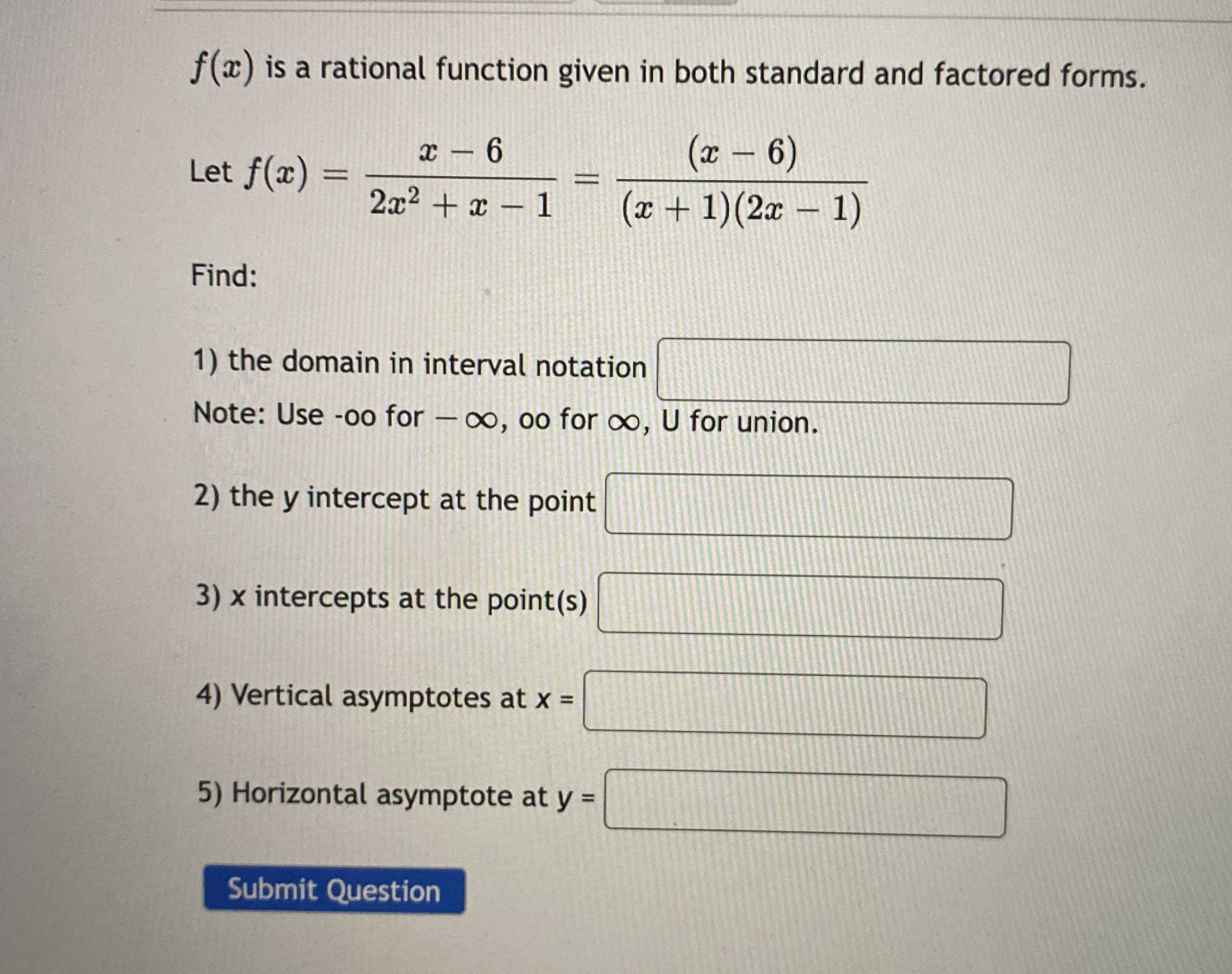### Still have math questions?

Algebra
Question$$f ( x )$$ is a rational function given in both standard and factored forms. Let $$f ( x ) = \frac { x - 6 } { 2 x ^ { 2 } + x - 1 } = \frac { ( x - 6 ) } { ( x + 1 ) ( 2 x - 1 ) }$$

Find: 1) the domain in interval notation 2) the $$y$$ intercept at the point 3) $$x$$ intercepts at the point(s) 4) Vertical asymptotes at $$x = \square$$ -oo for $$- \infty ,$$ oo for $$\infty , U$$ for union. 5) Horizontal asymptote at $$y = \square$$

Submit Question

1):$$(- \infty ,6)\cup (6,+ \infty )$$
3):-1,$$\frac{1}{2}$$